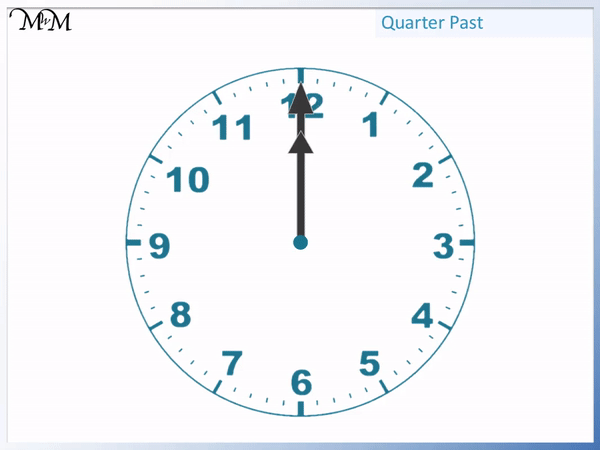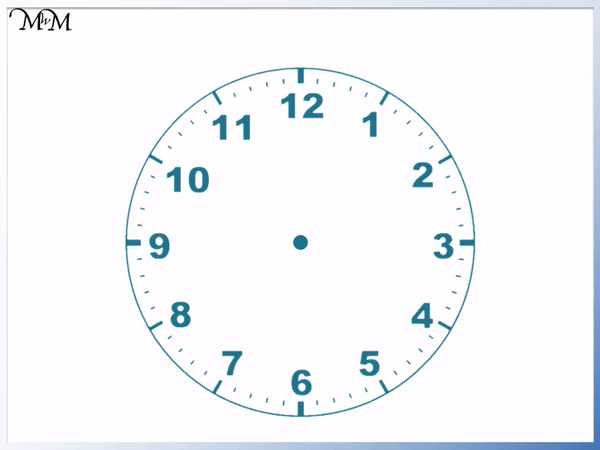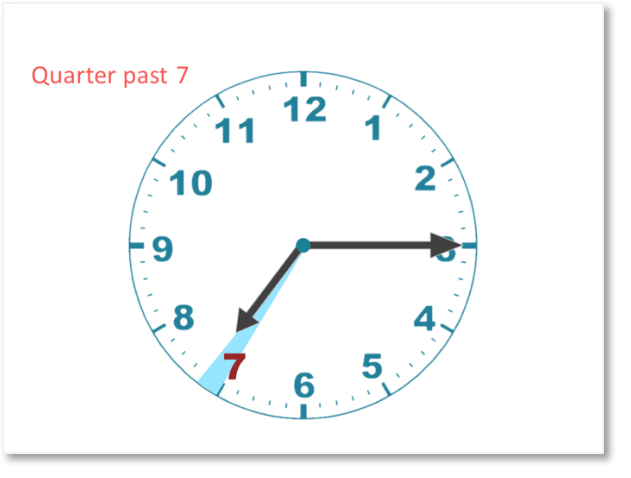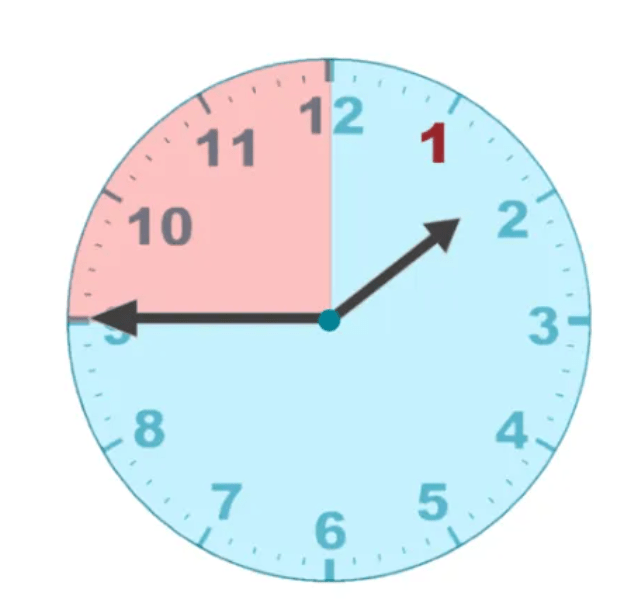# How to Tell the Time to Quarter Past the Hour

How to Tell the Time to Quarter Past the Hour• The longer minute hand completes a full turn in one hour.
• When the minute hand points at the 3, it has travelled a quarter of the way around the clock.
• When the minute hand points at the 3, we say that it is quarter past the hour.
• In this example, we start at one o’clock and the minute hand moves to point at the 3.
• The time shown is quarter past 1.
• We can see that the shorter hour hand has moved a quarter of the way from the 1 to the 2.

If the longer minute hand is pointing at the 3, then it is quarter past the hour.

To decide which hour, look at which number the shorter hour hand has just gone past.• The longer minute hand completes 7 cycles of the clock to get to 7 o’clock.
• The minute hand then continues to move until it is pointing at the 3.
• Because the minute hand is pointing at the 3, we know that it is quarter past the hour.
• We look at the shorter hour hand to decide which hour this is.
• The hour hand has gone past the 7 but has not yet reached the 8.
• So the time is quarter past 7.
• We can write this as 7:15 because one quarter of an hour is 15 minutes.Supporting Lessons

# Telling the Time to Quarter Past the Hour

## What is Quarter Past on a Clock?

Quarter past means that 15 minutes have passed since the last hour. There are 60 minutes in a whole hour and so, one-quarter of an hour is 15 minutes.

For example, if the time is ‘quarter past one’, then the time is 1:15 or 15 minutes past one.

The clock face contains two hands:

• The minute hand, which is the longer hand.
• The hour hand, which is the shorter hand.The minute hand completes one full turn in one hour. From one hour to the next, the minute hand moves from pointing at the 12 all the way around back to pointing at the 12 again.

In a quarter of an hour, the minute hand only gets one quarter of the way around the clock. It completes one quarter of a turn.

In the example below, we can see that the minute hand has moved one quarter of a turn. It is pointing at the 3.The time shown is one quarter past one.

Whenever the minute hand of a clock is pointing directly at the 3, the time is quarter past the hour. This is because there are 12 numbers on the whole clockface and 3 is one quarter of 12.

We can see that the shorter hour hand has moved one-quarter of the way from the 1 to the 2. It is still closer to the 1 than the 2 but it has gone past the 1.There are 60 minutes in a full hour. 60 ÷ 4 = 15 and so, one quarter of an hour is 15 minutes.

The time of ‘quarter past one’ can also be said as ‘one fifteen’ and it can be written as 1:15.## How to Show Quarter Past on a Clock

Quarter past the hour is shown with the longer minute hand pointing directly at the 3. To decide what hour this is, look at which number the shorter hour hand has just gone past.

For example, if the minute hand is pointing at the 3 and the hour hand has just gone past the 4 but not yet reached the 5, the time is quarter past 4.

Here is quarter past 4 shown on a clock.As soon as we see the minute hand pointing to the right, directly at the 3, we know that the time is quarter past the hour.

To decide what hour this is, we look at the hour hand to see what number it has just gone past.

When the time is quarter past the hour, the hour hand will not be pointing directly at a number. It will be in between two numbers. The smaller of these numbers is the hour that the time is a quarter past.Here we can see that the minute hand is pointing directly at the 3 but the hour hand is just past the 4. The time shown is a quarter past 4 or 4:15.

We can see that the hour hand has gone past the 4 but not yet past the 5. It cannot be a quarter past 5 yet. The hour hand is also closer to the 4 so the hour hand will be closest to pointing at the hour that it is a quarter past.

## How to Teach Quarter Past When Telling the Time

To teach time to quarter past the hour, first start by teaching how to read hours and half hours. Explain the pattern that the longer minute hand will always be pointing at the 3 whenever it is quarter past the hour. A ruler can be used to extend the hour hand so that you can easily read what hour it has just gone past.

When teaching quarter past, it is first necessary to understand how to read hours of the clock. It is also useful for a child to understand how long an hour is so that they have a reference for how much time has elapsed. To do this, you can tell your child when a new hour arrives, ask them to tell you when a new hour will arrive and also to predict what hour will come next.

It is also best to look at half hours before quarter hours as they can be easier to recognise.

Here is an example of the time of quarter past 7.It is helpful to show that the hour hand has moved a quarter of the way from the 7 towards the 8 as shown in the diagram.

It can also be helpful to show that the minute hand has moved a quarter of the way around the clock to get to the 3. You can cover the clock face with a circular piece of paper and then cut this paper into quarters. We can see that the quarter of the circle fits into the area where the minute hand has moved from the top to the 3. We can see this area shown on the clock below.This can help a child to understand why the time is called quarter past. They should first be familiar with the idea of shapes divided into quarters.Then as before, when it is quarter past the hour each day, it can be helpful to tell your child and ask them to let you know when it is quarter past an hour.

Here is the example of a quarter past twelve.

Here the minute hand is still pointing at the 3 and the hour hand has just moved past the 12.

This is an example worth teaching as the 12 is before the 1 and this can sometimes be confusing if not directly considered.Now try our lesson How to Read Quarter to the Hour on a Clock where we learn how to read quarter to the hour on a clock face.error: Content is protected !!